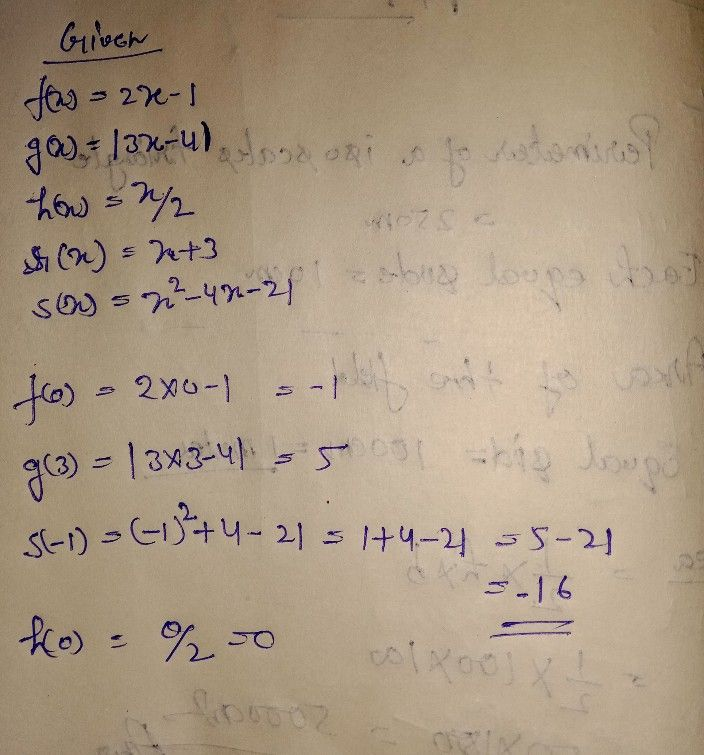Symbol
ProblemADDITIONAL ACTIVITY $Dircction$ Find out some of favorite punch lines by answering operations on functions problems below. Phrases of punch lines were coded by the letters of the correct answers. Write the punch lines on the lines provided. Given: $f\left(x\right)=2x-1$ $g\left(x\right)=|3x-4$ $h\left(x\right)=\dfrac {x} {2}$ r(x) $=x+3$ $s\left(x\right)=x^{2}-4x-21$ – – Column A Column B $-1f\left(0\right)=$ $A.-11$ 2. $g\left(3\right)=$ B. 2 3. $s\left(-1\right)=$ C. 3x + 2 4. h(0) = D. x 7 - 5. $r\right)\left(x\right)=$ E. $-x+4$ 6. ((f f +r)(3) = F. O = G. $2x^{2}+5x-3$ 7. $\left(r-f\right)\left(x$ 8. (r - f)(2) = H. 6 _9. $\left(f.r\right)\left(x$ = I. -16 10. (f •r)(1) = J. 2x + 2 _11. (x) = K. 5 _12. (-4) = L. 1 _13. $\left(ro0\left(x\right)$ = 14. $\left(r0D\left(2$ = MN0. . .4 -11 1 15. $\left(g°f\right)\left(1\right)=$
10th-13th grade
Probability and Statistics
Search count: 105
SolutionQanda teacher - henryseeStudent
Yung iba po?Qanda teacher - henrysee
dapat mong subukan kahit papaano ang kanilang mga sarili